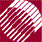ECE532 Biomedical Optics © 1998 Steven L. Jacques, Scott A. Prahl Oregon Graduate Institute

# Example: Mie calculation of Qs and µs

Consider the scattering of a HeNe laser beam by 0.304-µm-dia. nonabsorbing polystyrene spheres in water at a concentration of 0.1% volume fraction:

 np = 1.5721 particle refractive index nmed = 1.3316 medium refractive index a = 0.152 µm, particle radius= 0.6328 µm wavelength in vacuo fv = 0.001 volume fraction of particles in medium

### Calculate the following parameters:

refractive index mismatch ratio, size parameter, geometrical cross-sectional area, and number density

• nr = np/nmed = 1.5721/1.3316 = 1.1806

• x = 2πa/(/nmed) = (2)(3.1415)(0.152)/(0.6328/1.3316) = 2.0097

• A = πa2 = (3.1415)(0.152)2 = 0.0726 µm2

• ρs = fv/((4/3)πa3) = (0.001)/((4/3)(3.1415)(0.152)3) = 0.0608 µm-3

### Run the Mie theory algorithm:

• Mie(nr, x) ---> Qs

Mie(1.1806, 2.0097) ---> 0.1971

### Calculate the scattering coefficient:

• σs = QsA = (0.1971)(0.0726) = 0.01431 µm2

• µs = ρsσs = (0.0608)(0.01431) = 0.0009723 µm-1

• µs [cm-1] = (µs [µm-1])(104 [µm/cm]) = 9.723 cm-1